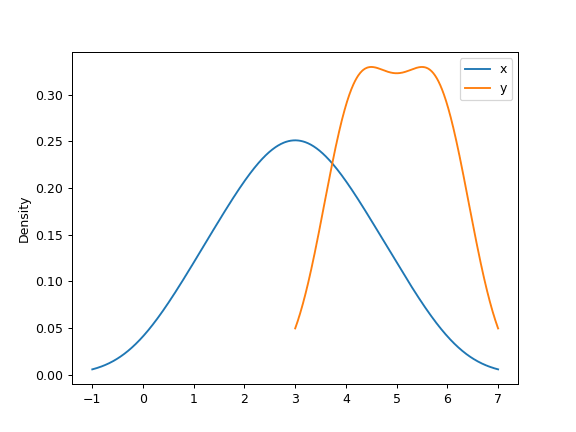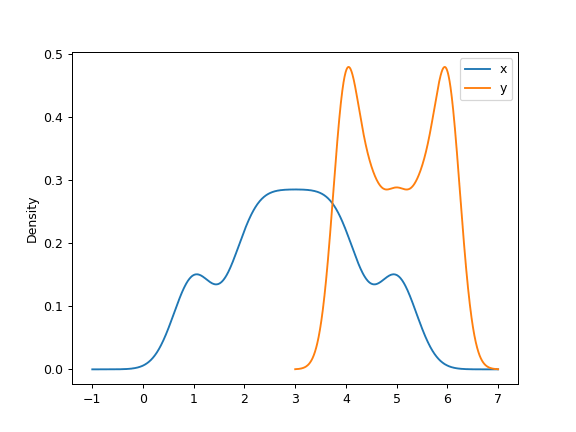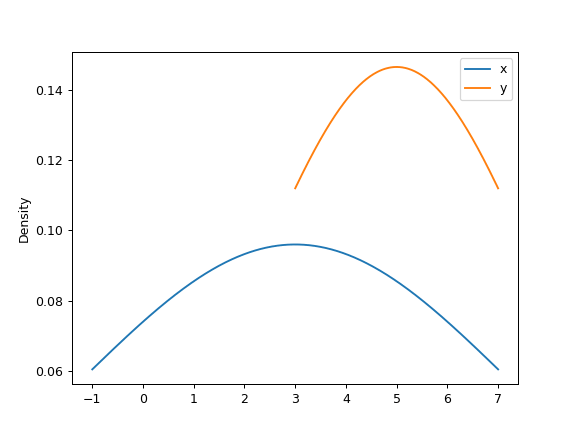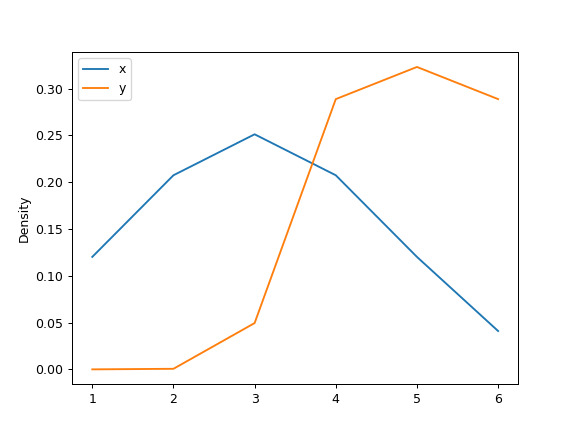/pandas 0.23

# pandas.DataFrame.plot.kde

`DataFrame.plot.kde(bw_method=None, ind=None, **kwds)` [source]

Generate Kernel Density Estimate plot using Gaussian kernels.

In statistics, kernel density estimation (KDE) is a non-parametric way to estimate the probability density function (PDF) of a random variable. This function uses Gaussian kernels and includes automatic bandwith determination.

Parameters: bw_method : str, scalar or callable, optional The method used to calculate the estimator bandwidth. This can be ‘scott’, ‘silverman’, a scalar constant or a callable. If None (default), ‘scott’ is used. See `scipy.stats.gaussian_kde` for more information. ind : NumPy array or integer, optional Evaluation points for the estimated PDF. If None (default), 1000 equally spaced points are used. If `ind` is a NumPy array, the KDE is evaluated at the points passed. If `ind` is an integer, `ind` number of equally spaced points are used. **kwds : optional Additional keyword arguments are documented in `pandas.DataFrame.plot()`. `axes : matplotlib.axes.Axes or numpy.ndarray of them`

See also

`scipy.stats.gaussian_kde`
Representation of a kernel-density estimate using Gaussian kernels. This is the function used internally to estimate the PDF.
`Series.plot.kde`
Generate a KDE plot for a Series.

#### Examples

Given several Series of points randomly sampled from unknown distributions, estimate their PDFs using KDE with automatic bandwidth determination and plot the results, evaluating them at 1000 equally spaced points (default):

```>>> df = pd.DataFrame({
...     'x': [1, 2, 2.5, 3, 3.5, 4, 5],
...     'y': [4, 4, 4.5, 5, 5.5, 6, 6],
... })
>>> ax = df.plot.kde()
```A scalar bandwidth can be specified. Using a small bandwidth value can lead to overfitting, while using a large bandwidth value may result in underfitting:

```>>> ax = df.plot.kde(bw_method=0.3)
``````>>> ax = df.plot.kde(bw_method=3)
```Finally, the `ind` parameter determines the evaluation points for the plot of the estimated PDF:

```>>> ax = df.plot.kde(ind=[1, 2, 3, 4, 5, 6])
```© 2008–2012, AQR Capital Management, LLC, Lambda Foundry, Inc. and PyData Development Team
Licensed under the 3-clause BSD License.
http://pandas.pydata.org/pandas-docs/version/0.23.4/generated/pandas.DataFrame.plot.kde.html